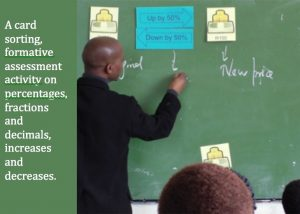This lesson is about different representations of number: percentages, decimals and fractions. It particularly emphasises that percentage and decimal increases and decreases are not mirrored whereas fraction increases and decreases are.

After a short introduction, during which the teacher asks students questions about amounts increasing or decreasing by 50%, 100% or 200%, the learners work in pairs (or small groups). They are given an A3 poster with 4 different amounts of money (one amount in each corner) as well as a small set of percentage increase and decrease arrow cards. Learners stick the arrows on the A3 poster to indicate the relationship between the different amounts of money. Once they have stuck down the percentage increase and decrease cards, hand out the decimal multiplier cards and then the fraction multiplier cards. After the activity the teacher holds a class discussion aiming to go over the answers and elicit something about the students’ understanding.

Note: This activity was designed as a formative assessment activity, in order to to provide the teacher with information about the learners’ current levels of understanding.

Tagged with: